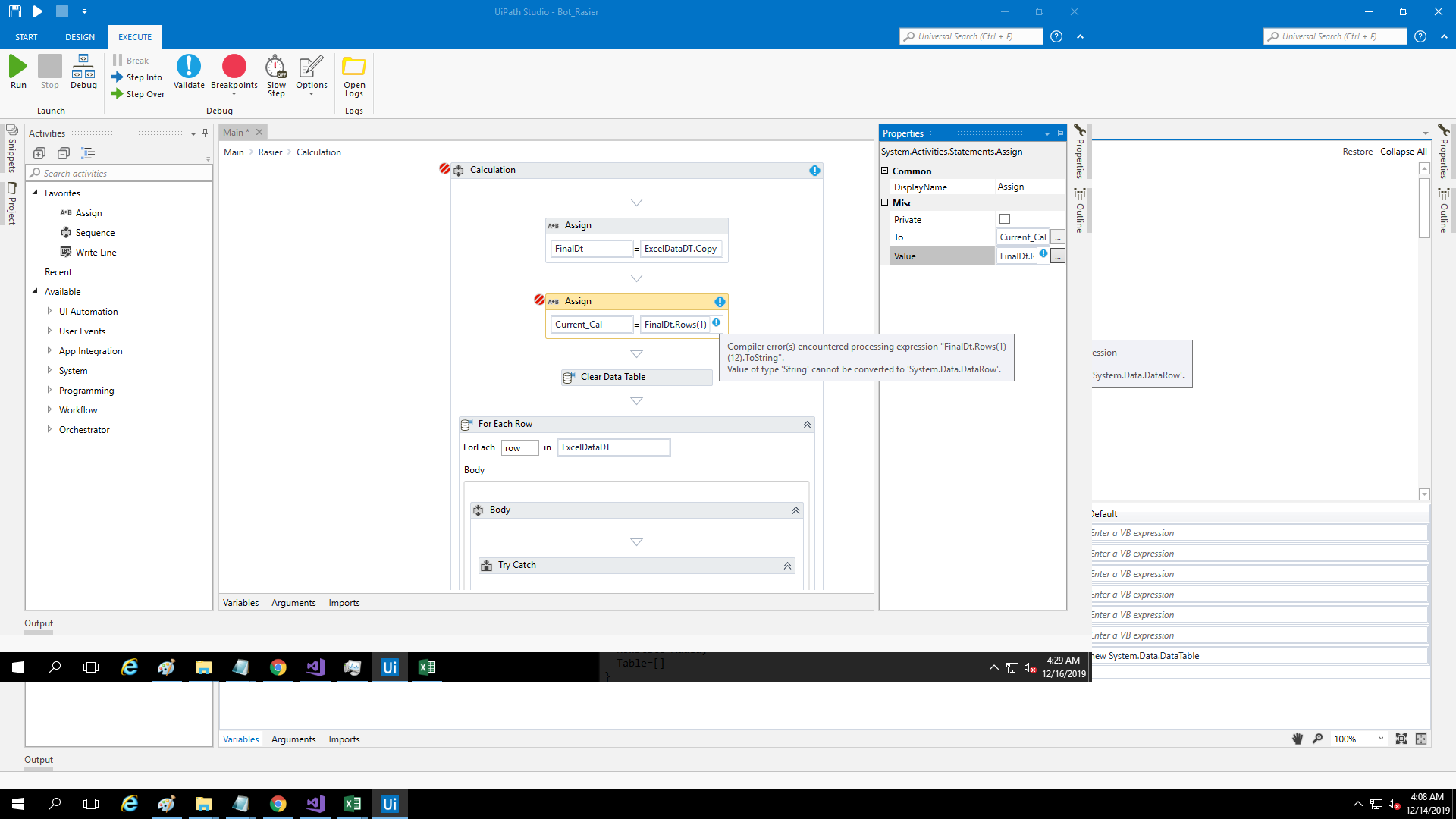# Error int converted to system.data.datarow[]

I getting error as mention in attachment.I copy datatabble data and assign new variable OF datatype datatable

1 Like

The generic type data type depends on the first variable or value given in the expression.

In your case, finalDt.Rows.IndexOf(11) will return the DataRow, so change_value1 data type is DataRow.

Here you are trying to add an integer to the data row. That’s why you are getting error.

Regards,
Karthik Byggari

I changed the variable to data row but still not work.As mentioned in attachment

Hi
The expression should be like this
FinalDt.Rows(indexvalue)

So
FinalDt.Rows(11)

This will give us a output of type Datarow at position 11 in that datatable

And if we want to add to that 11
It must be

FinalDt.Rows(11+1)
Or
Any int32 variable is also fine like this
FinalDt.Rows(11+int_var)

1 Like

Using this I am only got row FinalDt.Rows(11)
My requirement is I just want column value
eg I need in Firsr row 11 column value

1 Like

Then the expression be like this
FinalDt.Rows(0)(11).ToString

1 Like

yes I already try this got error1 Like

can you help me on this can we do like this
FinalDt.Select(“Name=‘GetFilePath’”)(0)(1).ToString

1 Like

FinalDt.Rows(0)(11).ToString
the syntax is datatable.Rows(rowindex)(columnindex) which will give us the value of that particular cell position in a datatable as both row index and column index starts from 0 for first row and first column
–being a particular value it will be type string
–as we are assigning a string value to a datarow variable Current_Cal its throwing error

if we need a particular row i.e datarow then the syntax is
yourdatatable.Rows(rowindex)
or
if we need particular row, column value then the syntax is
yourdatatable.Rows(rowindex)(columnindex)

based on your need we can choose among the two and

and for this
first use a assign activity like this
FinalDt = FinalDt.Select("[Name] = ‘GetFilePath’ ").CopyToDatatable()
then another assign activity like this
Current_Cal = FinalDt.Rows(0) \if we want to first row as output where Current_Cal is a datarow variable
Current_Cal = FinalDt.Rows(0)(11) \if we want the value at first row and 11 th column where Current_Cal is a string variable